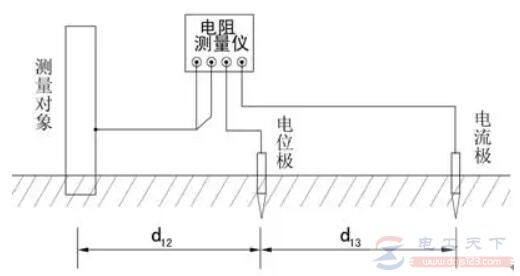# 牺牲阳极接地电阻值是多少

## 一、阳极接地电阻

Ra=ρln(L/r)/2πL

Ra=阳极接地电阻(ohms)

ρ=土壤电阻率(ohm-m)

L=阳极长度(m)

r=阳极半径（m）## 二、阳极驱动电位

V=阳极电位：高电位镁阳极-1.75V，低电位镁阳极-1.55V，锌阳极电位-1.10V。

## 四、应用举例

1、被保护面积：A=π×D×L

D=管道直径，159mm

L=管道长度，13x103m

A=3.14×0.159×13000=6490m2

2、所需阴极保护电流：I=A×Cd×（1-E）

I=阴极保护电流

Cd=保护电流密度，取10mA/m2

E=涂层效率，98%

I=6490×10×2%=1298mA

3、根据设计寿命以及阳极电容量计算阳极用量

W=8760It/ZUQ

I=阳极电流输出(Amps)

t=设计寿命(years)

U=电流效率(0.5)

Z=理论电容量(2200Ah/kg)

Q=阳极使用率（85%）

W=阳极重量(Kg)

W=8760×1.298×15/（2200×0.5×0.85）=183Kg

4、根据阳极实际发电量计算阳极用量

Ra=ρln(L/r)/2πL

Ra=阳极接地电阻(ohms)

ρ=土壤电阻率(ohm-m)

L=阳极长度(m)

r=阳极半径（m）

7.7Kg阳极填包后尺寸为：长=762mm，直径=152mm。

Ra=30×ln(2×0.762/0.152)/（2×3.14×0.762）=16.9Ω

5、牺牲阳极系统实际寿命验算：

t=WZUQ/8760I=30×7.7×2200×0.5×0.85÷（8760×1.298）=19

t=19years

`相关文章`